# NCERT Solutions for Class 8 Maths Chapter 5 Exercise 5.3

NCERT Solutions for Class 8 Maths Chapter 5 Exercise 5.3 – Data Handling, has been designed by the NCERT to test the knowledge of the student on the following topics :-

• Chance and Probability – Getting a result
• Chance and Probability – Equally likely outcomes
• Chance and Probability – Linking chances to probability
• Chance and Probability – Outcomes as events
• Chance and Probability – Chance and probability related to real life

### NCERT Solutions for Class 8 Maths Chapter 5 Exercise 5.3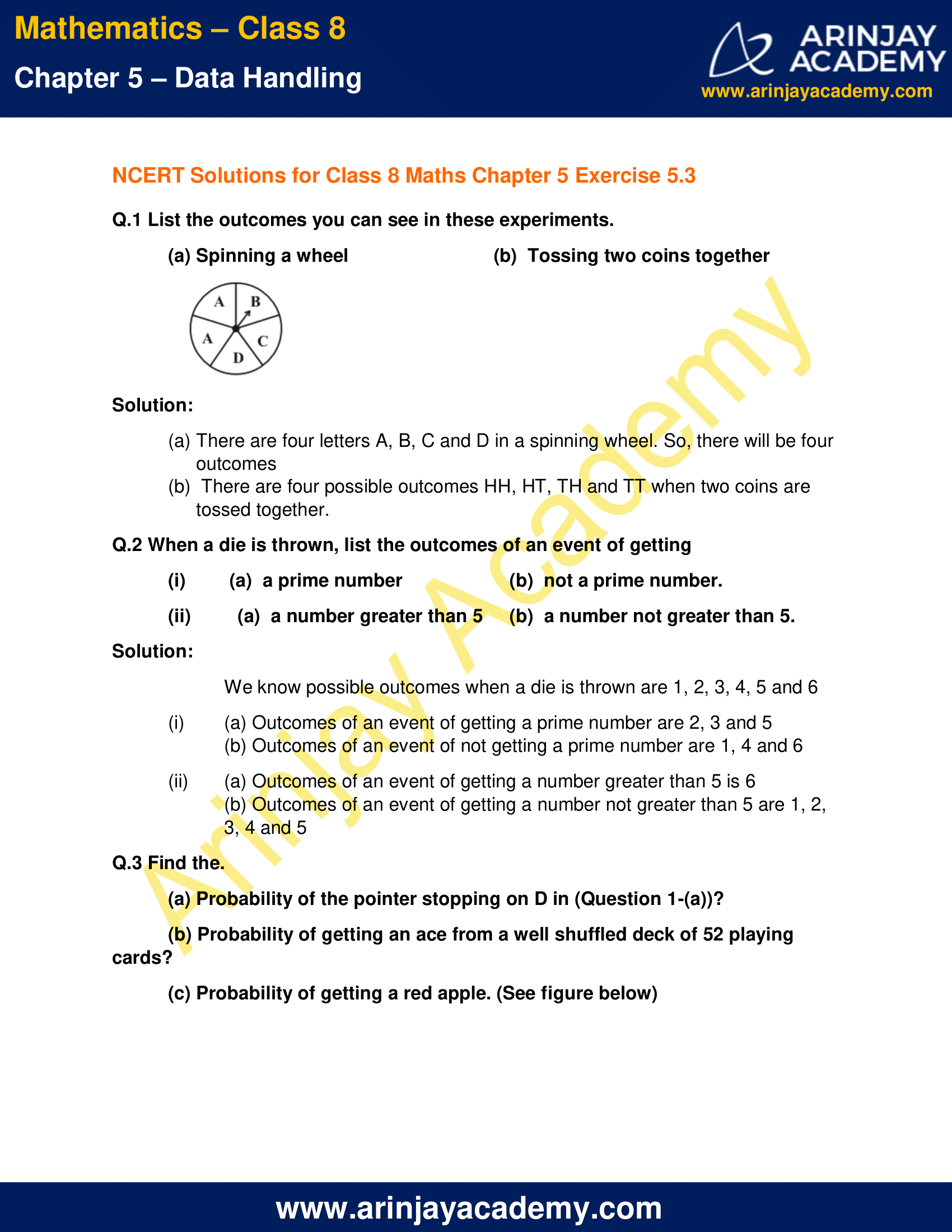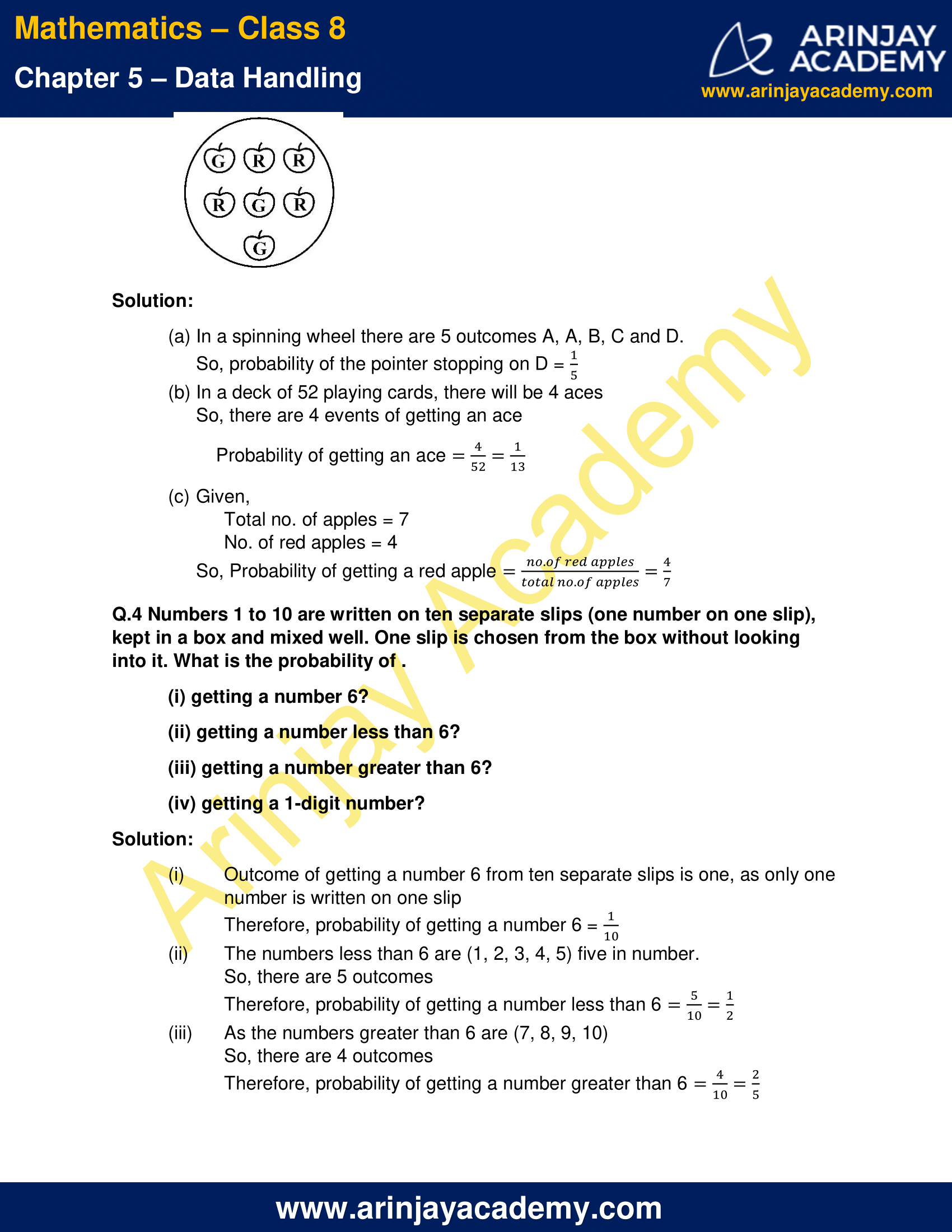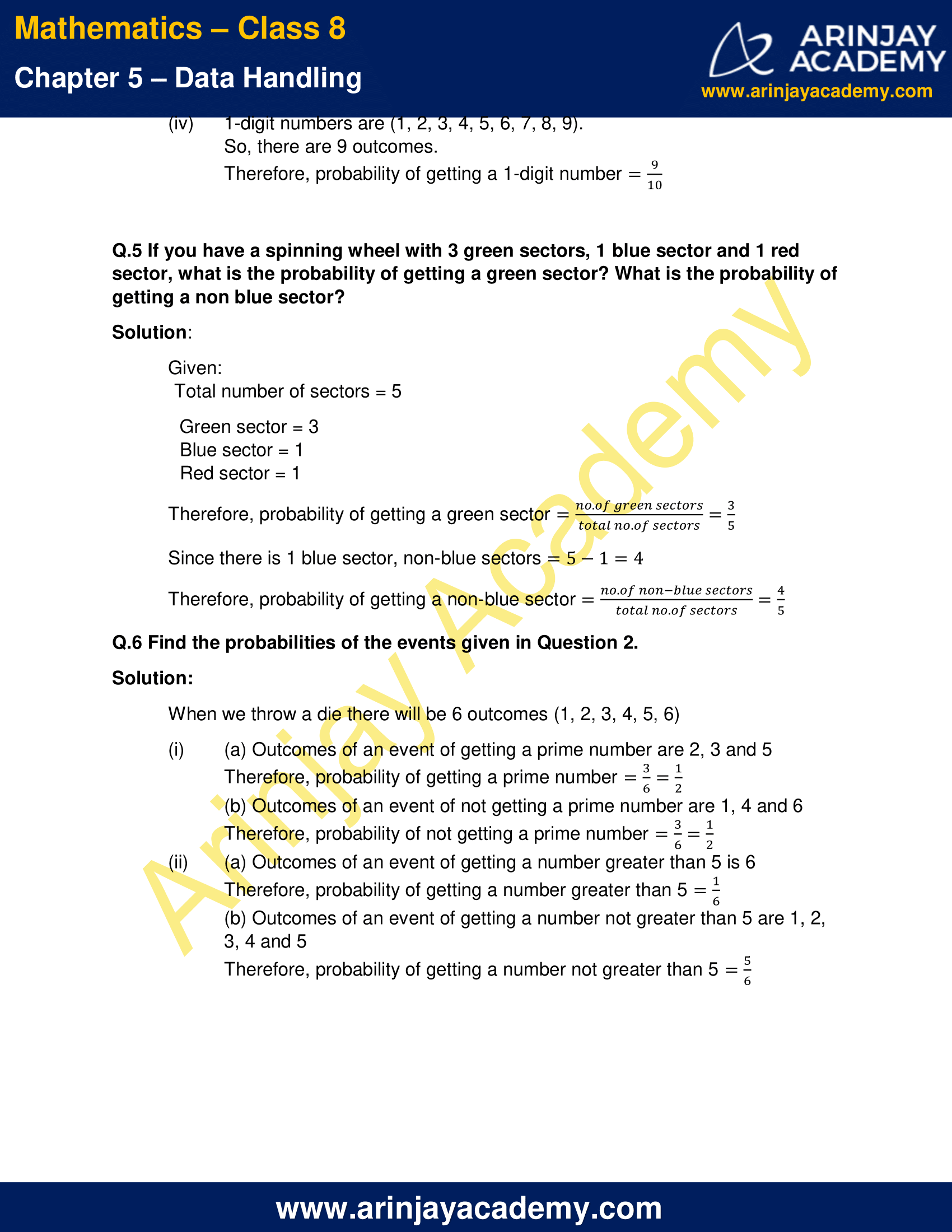NCERT Solutions for Class 8 Maths Chapter 5 Exercise 5.3

Q.1 List the outcomes you can see in these experiments.

(a) Spinning a wheel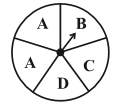(b)  Tossing two coins together

Solution:

(a) There are four letters A, B, C and D in a spinning wheel. So, there will be four outcomes
(b) There are four possible outcomes HH, HT, TH and TT when two coins are tossed together.

Q.2 When a die is thrown, list the outcomes of an event of getting

(i) (a)  a prime number
(b)  not a prime number.

(ii) (a)  a number greater than 5
(b)  a number not greater than 5.

Solution:

We know possible outcomes when a die is thrown are 1, 2, 3, 4, 5 and 6

(i) (a) Outcomes of an event of getting a prime number are 2, 3 and 5
(b) Outcomes of an event of not getting a prime number are 1, 4 and 6

(ii) (a) Outcomes of an event of getting a number greater than 5 is 6
(b) Outcomes of an event of getting a number not greater than 5 are 1, 2, 3, 4 and 5

Q.3 Find the.

(a) Probability of the pointer stopping on D in (Question 1-(a))?
(b) Probability of getting an ace from a well shuffled deck of 52 playing cards?
(c) Probability of getting a red apple. (See figure below)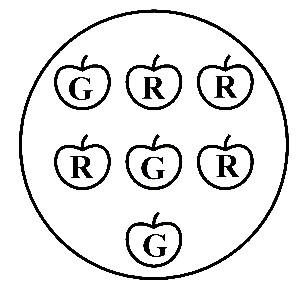Solution:

(a) In a spinning wheel there are 5 outcomes A, A, B, C and D.
So, probability of the pointer stopping on D = 1/5

(b) In a deck of 52 playing cards, there will be 4 aces
So, there are 4 events of getting an ace
Probability of getting an ace = 4/52 = 1/13

(c) Given,
Total no. of apples = 7
No. of red apples = 4
So, Probability of getting a red apple = (No. of red apples/Total no. of apples) = 4/7

Q.4 Numbers 1 to 10 are written on ten separate slips (one number on one slip), kept in a box and mixed well. One slip is chosen from the box without looking into it. What is the probability of .

(i) getting a number 6?
(ii) getting a number less than 6?
(iii) getting a number greater than 6?
(iv) getting a 1-digit number?

Solution:

(i) Outcome of getting a number 6 from ten separate slips is one, as only one number is written on one slip
Therefore, probability of getting a number 6 = 1/10

(ii) The numbers less than 6 are (1, 2, 3, 4, 5) five in number.
So, there are 5 outcomes
Therefore, probability of getting a number less than 6 = 5/10 = 1/2

(iii) As the numbers greater than 6 are (7, 8, 9, 10)
So, there are 4 outcomes
Therefore, probability of getting a number greater than 6 = 4/10 = 2/5

(iv) 1-digit numbers are (1, 2, 3, 4, 5, 6, 7, 8, 9).
So, there are 9 outcomes.
Therefore, probability of getting a 1-digit number = 9/10

Q.5 If you have a spinning wheel with 3 green sectors, 1 blue sector and 1 red sector, what is the probability of getting a green sector? What is the probability of getting a non blue sector?

Solution:

Given:
Total number of sectors = 5

Green sector = 3
Blue sector = 1
Red sector = 1

Therefore, probability of getting a green sector = (No. of green sectors/Total no. of sectors) = 3/5

Since there is 1 blue sector, non-blue sectors = 5 – 1 = 4

Therefore, probability of getting a non-blue sector = (No. of non-blue sectors/Total no. of sectors) = 4/5

Q.6 Find the probabilities of the events given in Question 2.

Solution:

When we throw a die there will be 6 outcomes (1, 2, 3, 4, 5, 6)

(i) (a) Outcomes of an event of getting a prime number are 2, 3 and 5
Therefore, probability of getting a prime number = 3/6 = 1/2

(b) Outcomes of an event of not getting a prime number are 1, 4 and 6
Therefore, probability of not getting a prime number = 3/6 = 1/2

(ii) (a) Outcomes of an event of getting a number greater than 5 is 6
Therefore, probability of getting a number greater than 5 = 1/6

(b) Outcomes of an event of getting a number not greater than 5 are 1, 2, 3, 4 and 5
Therefore, probability of getting a number not greater than 5 = 5/6

The next Exercise for NCERT Solutions for Class 8 Maths Chapter 6 Exercise 6.1 – Square and Square roots can be accessed by